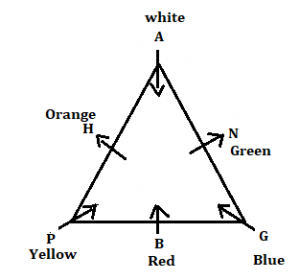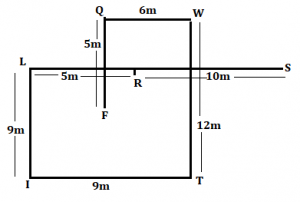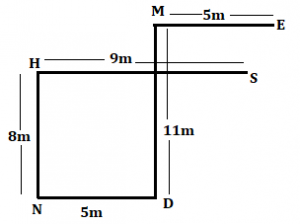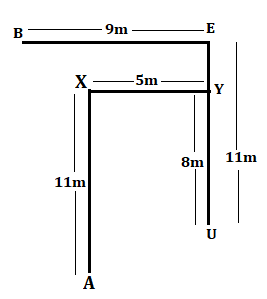Latest Banking jobs   »

# Reasoning Ability Quiz For SBI PO/Clerk Mains 2023- 13th January

Directions (1-5): Study the information carefully and answer the questions given below.
Six persons are sitting around a triangular table, in such a way three persons are sitting three corners while three are sitting at middle of the side. Some of them face towards center and some of them face outside the center. Each of them likes different colours i.e., Red, Blue, Green, Yellow, Orange and White.
A sits second to the right of G who likes Blue. One person sits between A and the one who likes yellow. Immediate neighbor of A face same direction to each other. B sits second to the right of N who likes green colour and both of them face opposite direction to each other. H sits second to the left of the one who likes red colour and both of them face opposite direction to each other. A is not immediate neighbor of the one who likes red. G does not sit immediate left of the one who likes red colour. Immediate neighbor of G does not face same direction to each other’s. The one who likes white colour face inside and P face inside. The one who likes orange face outside the center. A does not like Orange. The one who likes yellow colour doesn’t sit middle of the side.

Q1. Who among the following sits immediate right of the one who likes yellow?
(a) P
(b) B
(c) G
(d) H
(e) None of these

Q2. How many persons face outside the center?
(a) One
(b) Four
(c) Two
(d) Three
(e) None

Q3. How many persons sit between H and G when counted left of H?
(a) More than three
(b) Two
(c) Three
(d) One
(e) None

Q4. Who among the following person likes white?
(a) A
(b) B
(c) H
(d) P
(e) None of these

Q5. Who among the following sits third to the right of the one who likes green?
(a) The one who likes red
(b) The one who likes Orange
(c) The one who likes White
(d) The one who likes yellow
(e) None of these

Directions (6-10): In the following questions, the symbols #, %, \$, @ and © are used with the following meaning as illustrated below.
‘P # Q’ means ‘P is not smaller than Q’.
‘P % Q’ means ‘P is neither smaller than nor equal to Q’.
‘P \$ Q’ means ‘P is neither greater than nor equal to Q’.
‘P @ Q’ means ‘P is neither greater than nor smaller than Q’.
‘P © Q’ means ‘P is not greater than Q’.
Now, in each of the following questions assuming the given statements to be true, find which of the three Conclusions I, II and III given below them is/are definitely true and give your answer accordingly.

Conclusions: I. R%U
II. T#U
III. M%K
(a) Only I and III is true
(b) Only II and I is true
(c) Only II and III is true
(d) Only I is true
(e) None is true

Q7. Statements: D©G@ H, A%E#H, B\$D
Conclusions: I. B%A
II. E#D
III. G%E
(a) Only III and II is true
(b) Only II
(c) Only III is true
(d) Either I or III true
(e) None of these

Conclusions: I.Z%B
II. H@Z
III. G#A
(a) Only I is true
(b) I, II and III are true
(c) Either I or II true
(d) Only II is true
(e) None of these

Conclusions: I. L#Q
II. R%P
III. W%L
(a) Only II and III is true
(b) Only I is true
(c) Either I or III true
(d) Only II
(e) None of these

Q10. Statements: K%M@R, R#T%X, O\$X
Conclusions: I. M#T
II.R\$O
III. M%O
(a) Only I is true
(b) Only I and III is true
(c) Only II is true
(d) All are true
(e) None of these

Directions (11-13): Study the following information carefully and answer the questions given below.
P&Q (4)- P is 7m in north of Q
P%Q (8)- P is 11m in south of Q
P@Q (3)- P is 6m in east of Q
P#Q (9)- P is 12m in west of Q

S@R (7), W&T (9), I%L (6), L#R (2), T@I (6), Q&F (2), Q#W (3)

Q11. What is the direction and shortest distance of point W with respect to point S?
(a) 5m, South-west
(b) √45m, North-east
(c) 7m, South-East
(d) 6m, North
(e) None of these

Q12. What is the direction of point F with respect to point R?
(a) South
(b) South-east
(c) South-west
(d) North- east
(e) None of these

Q13. If Point A is 12m east of point F then what is the direction of A with respect to S?
(a) East
(b) North
(c) North east
(d) South
(e) None of these

Directions (14-15): Each of these questions is based on the following information:
(i) A & B means A is 8m North of B.
(ii) A % B means A is 5m West of B.
(iii) A * B means A is 9m East of B.
(iv) A @ B means A is 11m South of B.

Q14. If the expression ‘S*H&N%D@M%E’ is true, what is the shortest distance between M and H?
(a) √34m
(b) 6m
(c) 5m
(d) 7m
(e) None of these

Q15. If the expression ‘A@X%Y&U@E*B’ is true, what is the direction of Y with respect to B?
(a) East
(b) North east
(c) South West
(d) South east
(e) None of these

Solutions

Solutions (1-5):
Sol.S1. Ans. (b)
S2. Ans. (c)
S3. Ans. (b)
S4. Ans. (a)
S5. Ans. (d)

S6. Ans.(d)
Sol. I. R%U(true)
II. T#U(false)
III. M%K(false)

S7. Ans.(b)
Sol. I. B%A(false)
II. E#D(true)
III. G%E(false)

S8. Ans.(a)
Sol. I.Z%B(true)
II. H@Z(false)
III. G#A(false)

S9. Ans.(c)
Sol. I. L#Q(false)
II. R%P(false)
III. W%L(false)

S10. Ans.(b)
Sol. I. M#T(True)
II.R\$O(false)
III. M%O(True)

Solutions (11-13):
Sol.S11. Ans. (e)
S12. Ans. (c)
S13. Ans. (d)

S14. Ans. (a)
Sol.S15. Ans. (d)
Sol.## FAQs

### When is the SBI Clerk and PO Mains Exam 2022-23?

SBI Clerk & PO Mains exam are scheduled to be held on 15th & 30th January 2023.

#### Congratulations!